# The machine

The machine was in operation for 9 tenths (in a fraction) of eight hours of working time. How long has he been running?

Result

t =  7.2 h
t2 = 7:12 h### Step-by-step explanation: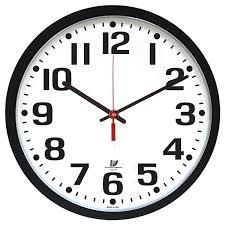Did you find an error or inaccuracy? Feel free to write us. Thank you!Math student
The quantity of six times 3 1/2 over 2.Math student
The quantity of six times 3 1/2 over 2

??
Convert 3 ½ into improper fraction.

3 ½ → 7/2

Convert into Multiplication Process

Lowest Term

= 10 ½Tips to related online calculators
Need help to calculate sum, simplify or multiply fractions? Try our fraction calculator.
Do you want to convert time units like minutes to seconds?

## Related math problems and questions:

• Fractions and mixed numerals(a) Convert the following mixed numbers to improper fractions. i. 3 5/8 ii. 7 7/6 (b) Convert the following improper fraction to a mixed number. i. 13/4 ii. 78/5 (c) Simplify these fractions to their lowest terms. i. 36/42 ii. 27/45 2. evaluate the follow
• Fraction unknowns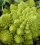Divide of fractions with unknowns: Fraction 1: The quantity x squared plus 6 times x plus 9 over the quantity x minus 1. Fraction 2: the quantity x squared minus 9 over the quantity x squared minus 2 times x plus 1.  Find Fraction 1 over Fraction 2.
• To improper fractionChange mixed number to improper fraction a) 1 2/15 b) -2 15/17
• Lowest terms 2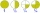The expression 4/12 can be expressed in its lowest term as 1/3. What is 3/15 expressed in its lowest term?
• Rajendra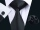Rajendra a farmer had two sons and two daughters. He decided to divide his property among his sons and daughters. So he wrote a "WILL" about distribution of his property. According to his "WILL", he desired to give 3/5 th of the property to his sons in eq
• Two looms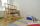On two different looms with different performance, the required quantity of textile in 6 hours when both work together. On the first machine, this quantity of the textile can be made in 10 hours. How long should it take to make the same quantity of textil
• Ingrid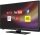Ingrid watched television for 2 1/4 hours, and Devon watched for 3 2/3 hours. For how many hours did they watch television in total?
• 5 2/55 2/5 hours a week  mathematics,  3 3/4 hours a week   Natural sciences, 4 3/8 hours a week  Technology . how many hours does  he spend on social sciences if he spend 17 1/2 hours a week for the four subject?
• The studyJames was preparing for a quiz. He studied 5/21 hours on Saturday and 2/7 hours on Sunday. How many hours did he study over the weekend?
• The average 3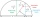The average of fraction is 7/10. One of the fractions is 2/3, the other fraction is?
• RunningJoanne ran 8 1/2 Kyleigh 1 2/3 and John 6 3/4 miles in one day. How many miles did the girls run in 3 days?
• Hours 3How many hours are in 3 2/3 days?
• The product 2The product of two functions is 10. If one of them is 2 1/3, find the other one.
• Evaluate mixed expressionsWhich of the following is equal to 4 and 2 over 3 divided by 3 and 1 over 2? A. 4 and 2 over 3 times 3 and 2 over 1 B. 14 over 3 times 2 over 7 C. 14 over 3 times 7 over 2 D. 42 over 3 times 2 over 31
• Jose studiedJose studied for 4 and 1/2 hours on Saturday and another 6 and 1/4 hours on Sunday. How many subjects did he study if he has alloted 1 and 1/2 hours per subject on Saturday and 1 and 1/4 hours per subject on Sunday?
• Guess a fractionTom was asked to guess a fraction. The sum of 1/2 the numerator and 1/3 of its denominator is 30. If Tom subtracts 36 from its denominator, the fraction becomes 1/3. What is the fraction that Tom was asked to guess? (Leave your answer in simplest form)
• PoolIf water flows into the pool by two inlets, fill the whole for 19 hours. The first inlet filled pool 5 hour longer than the second. How long pool take to fill with two inlets separately?